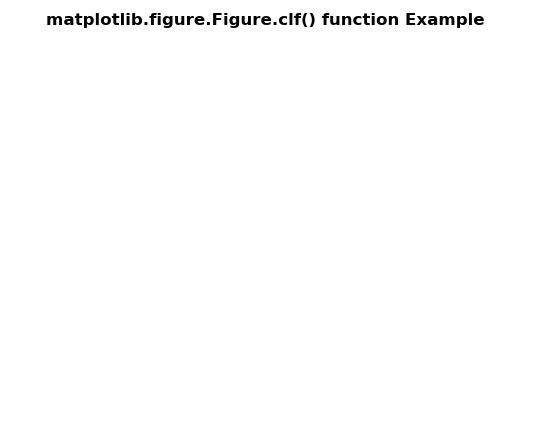Matplotlib.figure.Figure.clf() in Python

• Last Updated : 30 Apr, 2020

Matplotlib is a library in Python and it is numerical – mathematical extension for NumPy library. The figure module provides the top-level Artist, the Figure, which contains all the plot elements. This module is used to control the default spacing of the subplots and top level container for all plot elements.

matplotlib.figure.Figure.clf() function

The clf() method of figure module of matplotlib library is used to Clear the figure.

Syntax: clf(self, keep_observers=False)

Parameters: This accept the following parameters that are described below:

• keep_observers: This parameter is the boolean value.

Returns: This method does not return any value.

Below examples illustrate the matplotlib.figure.Figure.clf() function in matplotlib.figure:

Example 1:

 # Implementation of matplotlib functionimport numpy as npimport matplotlib.pyplot as plt      fig, ax = plt.subplots()  ax.set_xlabel('x-axis')ax.set_ylabel('y-axis')  ax.plot([1, 2, 3])ax.grid(True)  fig.clf(True)   fig.suptitle('matplotlib.figure.Figure.clf() \function Example\n\n', fontweight ="bold")  plt.show()

Output:Example 2:

 # Implementation of matplotlib functionimport numpy as npimport matplotlib.pyplot as plt      t = np.linspace(0.0, 2.0, 201)s = np.sin(2 * np.pi * t)   fig, [ax, ax1] = plt.subplots(2, 1, sharex = True)   ax.set_ylabel('y-axis')ax.plot(t, s)ax.grid(True)ax.set_title('Sample Example',             fontsize = 12,             fontweight ='bold')   ax1.set_ylabel('y-axis')ax1.plot(t, s)ax1.grid(True)  fig.clf(False)   fig.suptitle('matplotlib.figure.Figure.clf() \function Example\n\n', fontweight ="bold")  plt.show()

Output:Attention geek! Strengthen your foundations with the Python Programming Foundation Course and learn the basics.

To begin with, your interview preparations Enhance your Data Structures concepts with the Python DS Course. And to begin with your Machine Learning Journey, join the Machine Learning - Basic Level Course

My Personal Notes arrow_drop_up